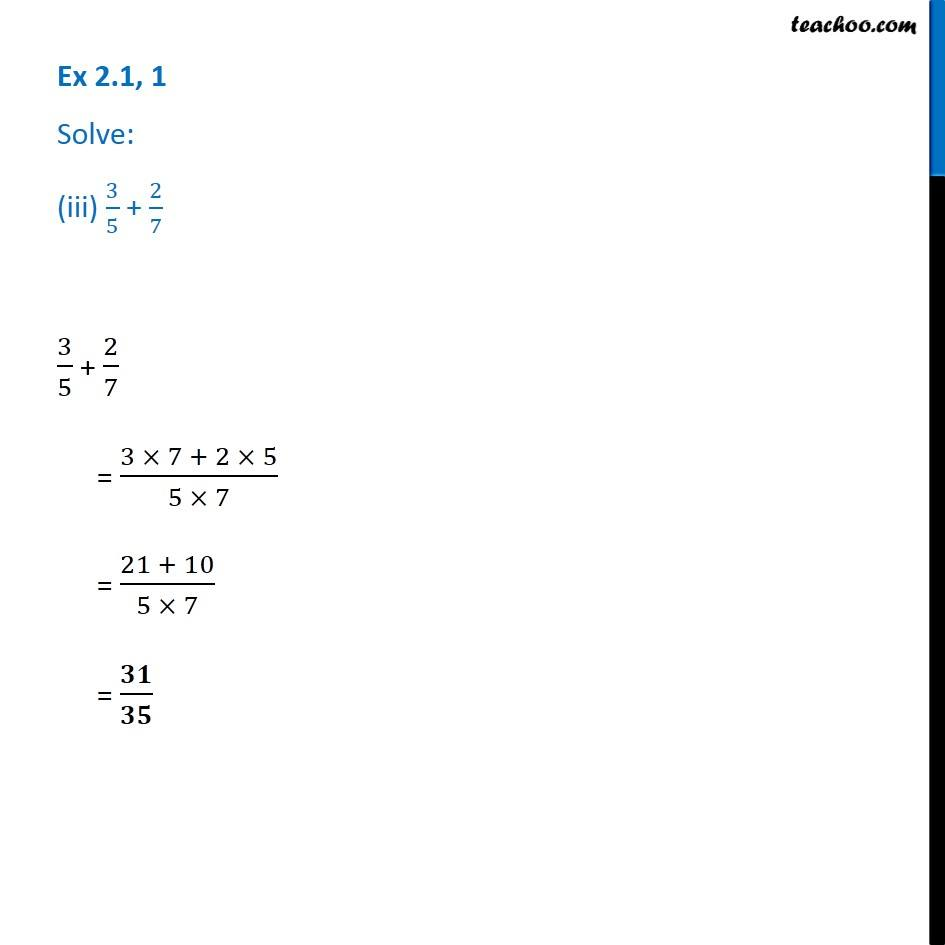Chapter 2 Class 7 Fractions and Decimals
Serial order wiseLearn in your speed, with individual attention - Teachoo Maths 1-on-1 Class

### Transcript

Question 1 Solve: (iii) 3/5 + 2/7 3/5 + 2/7 = (3 × 7 + 2 × 5)/(5 × 7) = (21 + 10)/(5 × 7) = 𝟑𝟏/𝟑𝟓 Question 1 Solve: (iv) 9/11 − 4/15 9/11 − 4/15 = (9 × 15 − 4 × 11)/(11 × 15) = (135 − 44)/165 = 𝟗𝟏/𝟏𝟔𝟓